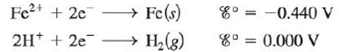Chapter 18, Problem 145CP

Chapter
Section
Textbook Problem

# A galvanic cell is based on the following half-reactions:where the iron compartment contains an iron electrode and [Fe2+] = 1.00 × 10-3 M and the hydrogen compartment contains a platinum electrode, P H 2 =   1.00  atm , and a weak acid, HA, at an initial concentration of 1.00 M. If the observed cell potential is 0.333 V at 25°C, calculate the Ka value for the weak acid HA.

Interpretation Introduction

Interpretation:

The half-reaction taking place in a galvanic cell having Hydrogen as a cathode with a weak acid HA in the cathode compartment. The value of Ka for the weak acid HA is to be calculated.

Concept introduction:

Dissociation constant (Ka) is used for measuring the strength of an acid. It is basically dissociation constant with reference to an acid and base that are in equilibrium with respect to each other.

To determine: The value of Ka for the given weak acid.

Explanation

Given,

The concentration of Fe2+ is 1.00×103M .

The pressure of Hydrogen gas is 1.00atm .

The concentration of weak acid HA is 1.00M .

The value of cell potential is 0.333V .

The reaction taking place at the cathode is,

2H++2eH2E°red=0.00V

The reaction taking place at the anode is,

Fe(s)Fe2++2eE°ox=0.440V

Add both the reduction and oxidation half-reactions as,

2H++2eH2(g)Fe(s)Fe2++2e

The final equation is,

2H++Fe(s)H2(g)+Fe2+

The value of E°cell is given as,

E°cell=E°ox+E°red

Where,

• E°ox is the oxidation potential of the electrode.
• E°red is the reduction potential of the electrode.

Substitute the values of E°ox and E°red in the above equation as,

E°cell=E°ox+E°red=0.440V+0.00V=0.440V_

The reaction involves the transfer of 2 moles of electrons.

The cell potential is calculated using the Nernst equation,

E=E°(0.0591n)log(Q)

Where,

• E is the cell potential.
• E° is the cell potential at the standard conditions.
• Q is the activity of the species in the cell.
• n is the number of electrons involved in the reaction.

Substitute the values of E° , Q, n , in the above equation as,

E=E°(0.0591n)log(Q)0.333=0.440(0.05912)log([Fe2+]pH2[H+]2)0.333=0.4400.02955log([1×103]1[H+]2)3.62=log([1×103]1[H+]2)

The equation is further simplified as,

3.62=log([1×103]1[H+]2)3

### Still sussing out bartleby?

Check out a sample textbook solution.

See a sample solution

#### The Solution to Your Study Problems

Bartleby provides explanations to thousands of textbook problems written by our experts, many with advanced degrees!

Get Started